# Lecture notes of the Nečas Center for Mathematical Modeling

## Lecture notes

Nečas Center for Mathematical Modeling is a collaborative effort between the Faculty of Mathematics and Physics of the Charles University, the Institute of Mathematics of the Academy of Sciences of the Czech Republic and the Faculty of Nuclear Sciences and Physical Engineering of the Czech Technical University.

One of the basic aims of the Nečas Center for Mathematical Modeling is to establish a scientific team studying mathematical properties of models in continuum mechanics and thermodynamics and to promote collaboration between members of the team and world renowned scientists. Another aim of the Nečas Center for Mathematical Modeling is to initiate Czech researchers to study new mathematical methods. To this end the experts, with research fields connected to the scientific program of the Nečas Center, are invited to present courses. The goal of the Lecture Notes of the Nečas Center for Mathematical Modeling is to record these courses.

### Editorial board

 Michal Beneš Department of Mathematics, Faculty of Nuclear Science and Physical Engineering, Czech Technical University, Prague, Czech Republic Pavel Drábek Center of Applied Mathematics, University of West Bohemia, Plzeň, Czech Republic Eduard Feireisl Mathematical Institute, Academy of Sciences of the Czech Republic, Prague, Czech Republic Miloslav Feistauer Department of Numerical Mathematics, Faculty of Mathematics and Physics, Charles University, Prague, Czech Republic Josef Málek Mathematical Institute, Faculty of Mathematics and Physics, Charles University, Prague, Czech Republic Jan Malý Department of Mathematical Analysis, Faculty of Mathematics and Physics, Charles University, Prague, Czech Republic Šárka Nečasová Mathematical Institute, Academy of Sciences of the Czech Republic, Prague, Czech Republic Jiří Neustupa Mathematical Institute, Academy of Sciences of the Czech Republic, Prague, Czech Republic Antonín Novotný Laboratoire d'Analyse Non Linéaire Appliquée et Modélisation, Institut de Mathematiques de Toulon, Université Toulon et du Var, Toulon, France K. R. Rajagopal Department of Mechanical Engineering, Texas A&M University, College Station, Texas, United States of America Hans-Georg Roos Institute of Numerical Mathematics, Department of Mathematics, Technical University of Dresden, Dresden, Germany Tomáš Roubíček Mathematical Institute, Faculty of Mathematics and Physics, Charles University, Prague, Czech Republic Daniel Ševčovič Department of Applied Mathematics and Statistics, Faculty of Mathematics, Physics & Informatics, Comenius University, Bratislava, Slovakia Vladimír Šverák School of Mathematics, University of Minnesota, Minneapolis, Minnesota, United States of America

### Managing editors

Petr Kaplický, kaplicky@karlin.mff.cuni.cz
Vít Průša, prusv@karlin.mff.cuni.cz

## Instructions for authors

Instructions for authors contributing to the Lecture notes are available here.

## Published volumes

### Product integration, its history and applications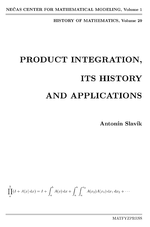Volume Product integration, its history and applications is devoted to the theory of product integral, its history and applications. The volume was published jointly with Department of Mathematics Education in series History of Mathematics.

• Chapter 1. Introduction
• Chapter 2. The origins of product integration
• Chapter 3. Lebesgue product integration
• Chapter 4. Operator-valued functions
• Chapter 5. Product integration in Banach algebras
• Chapter 6. Kurzweil and McShane product integrals
• Chapter 7. Complements

### Reviews in geomechanics

Reviews in Geomechanics (Lecture notes from Workshop on Geomaterials held in the Center in September 2006).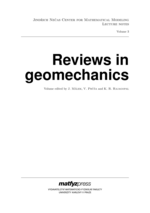• Part 1. Modeling of asphalt
• Chapter 1. The physical and chemical structure of asphalt: with a brief history of their usage and availability (J. Murali Krishnan, K. R. Rajagopal)
• Chapter 2. The mechanical behavior of asphalt (J. Murali Krishnan)
• Chapter 3. Internal structure and modeling of asphalt mixtures (Eyad Massad)
• Part 2. Soil and granular materials
• Chapter 1. Interface behaviour of soils (R. G. Robinson)
• Chapter 2. Consolidation behaviour of reclamation fill made of lumps (R. G. Robinson)
• Chapter 3. Modelling the behaviour of soils and granular materials (I. F. Collins)
• Part 3. Hypoelasticity and viscoelasticity
• Chapter 1. Hypoelasticity (B. Bernstein)
• Chapter 2. Viscoelasticity (B. Bernstein)

### Topics on partial differential equations

Topics on partial differential equations (lecture notes from various courses given in academic year 2006/2007 by guests of the Center).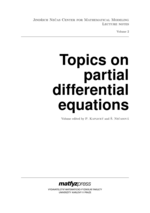• Part 1. Very weak, weak and strong solutions to the instationary Navier--Stokes system (Reinhard Farwig, Hideo Kozono, Hermann Sohr)
• Chapter 1. Introduction
• Chapter 2. Theory of very weak solutions
• Chapter 3. Regularity of weak solutions
• Part 2. Qualitative and quantitative aspects of curvature driven flows (Daniel Ševčovič)
• Chapter 1. Introduction
• Chapter 2. Preliminaries
• Chapter 3. Qualitative behavior of solutions
• Chapter 4. Level set methods for curvature driven flows of planar curves
• Chapter 5. Numerical methods for the direct approach
• Chapter 6. Applications of curvature driven flows
• Part 3. The Navier--Stokes equations with particle methods (Werner Varnhorn)
• Chapter 1. The Navier--Stokes equations with particle methods
• Part 4. Qualitative theory of semilinear parabolic equations and systems (Pavol Quittner)
• Chapter 1. Qualitative theory of semilinear parabolic equations and systems
• Part 5. Nonlinear Fredholm operators and PDEs (Patrick J. Rabier)
• Chapter 1. Degree for Fredholm mappings of index 0
• Chapter 2. Fredholmness and properness of differential operators

### Topics in mathematical modeling

Topics in mathematical modeling (lecture notes from various courses given in academic year 2007/2008 by guests of the Center).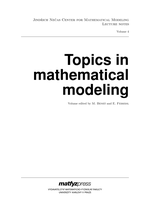• Part 1. (Masato Kimura) Shape derivative of minimum potential energy: abstract theory and applications
• Chapter 1. Shape derivative of minimum potential energy: abstract theory and applications
• Part 2. (Masato Kimura) Geometry of hypersurfaces and moving hypersurfaces in ${R}^m$ for the study of moving boundary problems
• Chapter 1. Preliminaries
• Chapter 2. Differential calculus on hypersurfaces
• Chapter 3. Signed distance function
• Chapter 4. Curvilinear coordinates
• Chapter 5. Moving hypersurfaces
• Chapter 6. Variational formulas
• Chapter 7. Gradient structure and moving boundary problems
• Part 3. Large time behaviour for diffusive Hamilton-Jacobi equations (Philippe Laurençot)
• Chapter 1. Introduction
• Chapter 2. Well-posedness and smoothing effects
• Chapter 3. Extinction in finite time
• Chapter 4. Temporal decay estimates for integrable initial data: $\sigma=1$
• Chapter 5. Temporal growth estimates for integrable initial data: $\sigma=-1$
• Chapter 6. Convergence to self-similarity
• Part 4. An area-preserving crystalline curvature flow equation: introduction to mathematical aspects, numerical computations, and a modeling perspective (Shigetoshi Yazaki)
• Chapter 1. An area-preserving crystalline curvature flow equation: introduction to mathematical aspects, numerical computations, and a modeling perspective

### Qualitative properties of solutions to partial differential equations

Qualitative properties of solutions to partial differential equationsl (lecture notes from various courses given in academic year 2007/2008 by guests of the Center).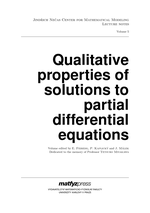The volume contains lecture notes from various courses given in academic year 2007/2008 and 2008/2009 by guests of the Center, the list of cotributions is the following:

• Part 1. The rugosity effect (Dorin Bucur)
• Chapter 1. Some classical examples
• Chapter 2. Variational analysis of the rugosity effect
• Part 2. Nonlinear evolution equations with anomalous diffusion (Grzegorz Karch)
• Chapter 1. Lévy operator
• Chapter 2. Fractal Burgers equation
• Chapter 3. Fractal Hamilton-Jacobi-KPZ equations
• Chapter 4. Other equations with Lévy operator
• Part 3. On a continuous deconvolution equation for turbulence models (Roger Lewandovski)
• Chapter 1. Introduction and main facts
• Chapter 2. Mathematical tools
• Chapter 3. From discrete to continuous deconvolution operator
• Chapter 4. Application to the Navier-Stokes equations
• Part 4. Rough boundaries and wall laws (Andro Mikelić)
• Chapter 1. Rough boundaries and wall laws
• Part 5. Hyperbolic problems with characteristic boundary (Alessandro Morando, Paolo Secchi, Paola Trebeschi)
• Chapter 1. Introduction
• Chapter 2. Compressible vortex sheets
• Chapter 3. An example of loss of normal regularity
• Chapter 4. Regularity for characteristic symmetric IBVPs

### Anisotropic function spaces and maximal regularity for parabolic problems (Part 1: Function spaces)

Anisotropic function spaces and maximal regularity for parabolic problems (Part 1: Function spaces) (First part of Herbert Amann's treatise on maximal regularity for linear parabolic systems.)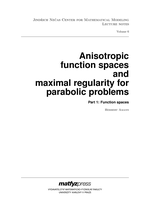This work contains a detailed and widely extended version of a series of lectures on maximal regularity theory presented at the Jindřich Nečas Center for Mathematical Modeling in Prague, in October 2007.

• Introduction
• Chapter 1. Multiplier estimates
• Chapter 2. Anisotropic Banach scales
• Chapter 3. Fourier multipliers and function spaces
• Chapter 4. Distributions on half-spaces and corners

### Topics in mathematical modeling and analysis

Topics in mathematical modeling and analysis (lecture notes from various courses given in academic year 2010/2011 by guests of the Center).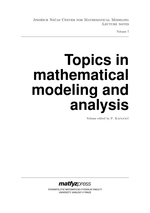• Part 1. Weighted Sobolev spaces and elliptic problems in the half-space (Chérif Amrouche)
• Chapter 1. Weighted Sobolev spaces and elliptic problems in the half-space
• Part 2. The control of PDEs (Enrique Fernández-Cara}
• Chapter 1. Optimal control of systems governed by PDEs
• Chapter 2. Controllability of the linear heat and wave PDEs
• Chapter 3. Controllability results for other time-dependent PDEs
• Part 3. Generalised trigonometric functions, compact operators and the $p$-Laplacian (David E. Edmunds)
• Chapter 1. Generalised trigonometric functions, compact operators and the $p$−Laplacian
• Part 4. Fluid-structure interaction for shear-dependent non-Newtonian fluids (Anna Hundertmark-Zaušková, Maria Lukáčová-Medvid'ová, Gabriela Rusnáková)
• Chapter 1. Fluid-structure interaction for shear-dependent non-Newtonian fluids
• Part 5. Analysis in Orlicz spaces (Ron Kerman)
• Chapter 1. Analysis in Orlicz spaces
• Part 6. Topics in the Q-tensor theory of liquid crystals (Arghir Zarnescu)
• Chapter 1. Mathematical modelling
• Chapter 2. Qualitative features of a stationary problem: Oseen--Frank limit
• Chapter 3. Well-posedness of a dynamical model: Q-tensors and Navier--Stokes

## Volumes in preparation

We are preparing the second part of Herbert Amann's treatise on maximal regularity for linear parabolic systems.